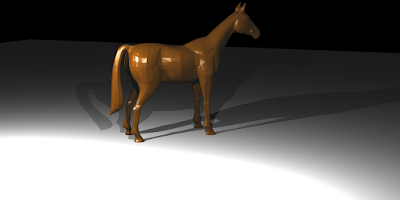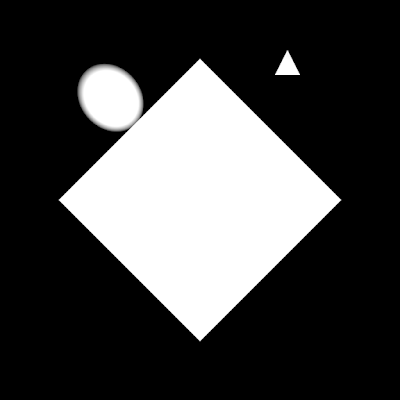## Thursday, March 16, 2017

### Assignment 2: Instancing and Smooth Triangles

Hello again. We are required implement smooth triangles and object instances for this assignment. Smooth triangles make use of three normal vectors, per-vertex, instead of using just a single normal vector for the whole triangle. By means of barycentric coordinates computed for the intersection point and these normal vectors, we can have better visuals. The outputs show this clearly.

Another issue was to implement instancing. Instead of having different memory locations for every instance of mesh, I preferred to use an instance class such that every single instance uses the same vertices but with different transformation matrices. Briefly, we do not transform the vertices but we apply the inverse transformation to the ray. This is more practical in general and when it comes to spheres, it eases our work.

Here are the outputs:output of horse.xml kernel execution time: 3.54 secondsoutput of horse_instanced.xml kernel execution time: 10.48 secondsoutput of simple_transform.xml kernel execution time: 2.92 millisecondsoutput of spheres_transform.xml kernel execution time: 3.32 milliseconds
Lessons learned:
1. I used -use_fast_math in the command line of the nvcc compiler. This command makes nvcc to optimize some of the functions and arithmetic operations by using some intrinsics which approximates the results. This command speeded up my ray tracer up to 1.25x. You can find the details of it in the related section of CUDA C Programming Guide.
2. Occupancy is one of the most important concept when you are dealing with CUDA. It basically states the ratio of number of active warps(a warp contains 32 threads) to the number of possible active warps(device limit). However, %100 occupancy does not always mean that it gives the best results. For example, in my case, the number of registers per multiprocessor was the limiting case. If I set it to 32(65536 registers per multiprocessor/2048 active threads per multiprocessor) registers per thread, occupancy increases to %100 but threads use a limited amount of registers. If I let the nvcc decides, it uses 48 registers per thread which gives a poor occupancy. I manually set it to 36 and got the best results. However, it might always change.
3. CUDA featured graphics cards do not have branch predictors. Using branches in your code is not the end of the life. However, if you use it unnecessarily or you put large amount of code(or function that contains) to different execution paths in the same warp, you will suffer from divergence. It is explained neatly in the CUDA C Best Practices Guide
4. Beside CUDA related issues, there was one thing that made me busy: instancing implementation for the spheres. After we transform our rays to object space by applying the inverse transformation of the object, we calculate the distance parameter in that space. Our professor told us that we can compare and use this parameter with other parameters calculated in other object spaces or in world space. This worked very well for triangles but I was suspicious if it is working for spheres or not. However, here, Matt Phar verifies that it works for the spheres as well. The only difference is that I was using geometric approach for ray-sphere intersection but they use the analytic solution. After some drawing and calculations on the paper, I understood that it is not possible to use distance parameter calculated in the object space in anywhere else if you are using geometric approach. Finally, I adopted the analytic solution for the ray-sphere intersection. Keep in mind that you should not normalize the direction vector of the transformed ray. If you do that, you cannot make use of what I've just explained above. The reason for this is well-explained in the last link.